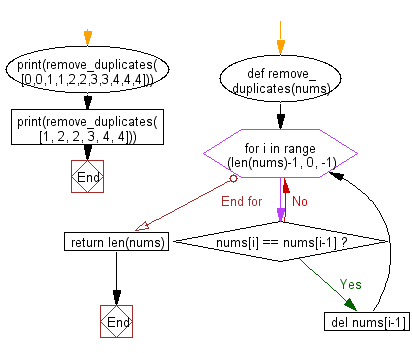﻿ Python: Remove the duplicate elements of a given array of numbers - w3resource# Python: Remove the duplicate elements of a given array of numbers

## Python Basic - 1: Exercise-73 with Solution

Write a Python program to remove the duplicate elements of a given array of numbers such that each element appear only once and return the new length of the given array.

Sample Solution:

Python Code:

``````def remove_duplicates(nums):
for i in range (len(nums)-1, 0, -1):
if nums[i] == nums[i-1]:
del nums[i-1]
return len(nums)

print(remove_duplicates([0,0,1,1,2,2,3,3,4,4,4]))
print(remove_duplicates([1, 2, 2, 3, 4, 4]))
``````

Sample Output:

```5
4
```

Pictorial Presentation:Flowchart:Python Code Editor:

Have another way to solve this solution? Contribute your code (and comments) through Disqus.

What is the difficulty level of this exercise?

Test your Programming skills with w3resource's quiz.

﻿

## Python: Tips of the Day

What is the difference between Python's list methods append and extend?

append: Appends object at the end.

```x = [1, 2, 3]
x.append([4, 5])
print (x)
```

Output:

```[1, 2, 3, [4, 5]]
```

extend: Extends list by appending elements from the iterable.

```x = [1, 2, 3]
x.extend([4, 5])
print (x)
```

Output:

```[1, 2, 3, 4, 5]
```

Ref: https://bit.ly/2AZ6ZFq